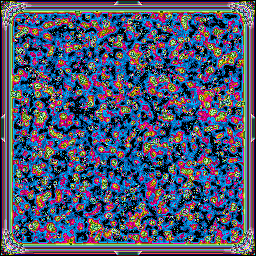• Home
• Files
• But thus do I counsel you, my friends: distrust all in whom the impulse to punish is powerful!

# Day 12

Previous: Day 11 | Next: Day 13
###AUTO-ACQUIRED DATA FOLLOWS…
archivist/day12.lisp
archivist/day12.lisp
```(asdf:load-system :split-sequence)
(defpackage \#:aoc-12
(:use \#:cl \#:split-sequence \#:iterate \#:alexandria))
(in-package \#:aoc-12)

(defun getinput ()
(mapcar (lambda (x)
(list (coerce (subseq x 0 1)
&\#39;character)
(parse-integer (subseq x 1))))
in)))

(defstruct ship
(x 0)
(y 0)

(defun rot (ship d)
360)))

(defun f (ship val)
(let ((px (round (\* val
(cos (\* pi (/ (ship-heading ship) 180))))))
(py (round (\* val
(sin (\* -1 pi (/ (ship-heading ship) 180)))))))
(move ship px py)))

(defun move (ship x y)
(setf (ship-x ship)
(+ (ship-x ship)
x))
(setf (ship-y ship)
(+ (ship-y ship)
y)))

(defun intcmd (ship cmd)
(cond
((eq (car cmd) \#\N)
((eq (car cmd) \#\S)
(move ship 0 (\* -1 (cadr cmd))))
((eq (car cmd) \#\E)
((eq (car cmd) \#\W)
(move ship (\* -1 (cadr cmd)) 0))
((eq (car cmd) \#\R)
((eq (car cmd) \#\L)
(rot ship (\* -1 (cadr cmd))))
((eq (car cmd) \#\F)

))

(defun part1 ()
(let\* ((in (getinput))
(s (make-ship)))
(iter (while in)
(let ((c (pop in)))
(intcmd s c))
(print s))
(+ (abs (ship-x s))
(abs (ship-y s)))))

(defstruct wpt
(x 10)
(y 1))

(defun wrot (pt val)
(let\* ((ang (\* pi (/ val 180)))
(oldx (wpt-x pt)))
(setf (wpt-x pt)
(+ (\* (round (cos ang)) (wpt-x pt))
(\* (round (sin ang)) (wpt-y pt))))
(setf (wpt-y pt)
(+ (\* (round (sin (\* -1 ang))) oldx)
(\* (round (cos ang)) (wpt-y pt))))))

(defun wmove (pt x y)
(setf (wpt-x pt)
(+ (wpt-x pt)
x))
(setf (wpt-y pt)
(+ (wpt-y pt)
y)))
(defun goto (ship pt val)
(iter (repeat val)
(setf (ship-x ship)
(+ (ship-x ship)
(wpt-x pt)))
(setf (ship-y ship)
(+ (ship-y ship)
(wpt-y pt)))))

(defun wintcmd (ship pt cmd)
(cond
((eq (car cmd) \#\N)
((eq (car cmd) \#\S)
(wmove pt 0 (\* -1 (cadr cmd))))
((eq (car cmd) \#\E)
((eq (car cmd) \#\W)
(wmove pt (\* -1 (cadr cmd)) 0))
((eq (car cmd) \#\R)
((eq (car cmd) \#\L)
(wrot pt (\* -1 (cadr cmd))))
((eq (car cmd) \#\F)

(defun part2 ()
(let\* ((in (getinput))
(s (make-ship))
(p (make-wpt)))
(iter (while in)
(let ((c (pop in)))
(wintcmd s p c))
(print s)
(print p)
)
(+ (abs (ship-x s))
(abs (ship-y s)))))
```

Tags: